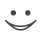# Get these flashcards, study & pass exams. For free! Even on iPhone/Android!

## Metric units (9 Cards)

How do we use a metric system?
If it is higher divide.
If it is lower multiply.
How many numbers are in a kilometer?
There are 1,000
How many numbers are in a hectometer?
There are 100
How many numbers are in Dekameter?
There are 10
How many numbers are in a meter?
There are 0
How many numbers are in  decimeters?
There are 10
How many numbers are in centimeters?
There are 100
How many numbers are in millimeters?
There 1000
Example:
KM to meter
5km times1000=5000Flashcard set info:
Author: hiphopari
Main topic: Mathematics
Topic: Metric units
Published: 24.02.2010
Tags: Math

Card tags:
All cards (9)
no tagsReport abuse